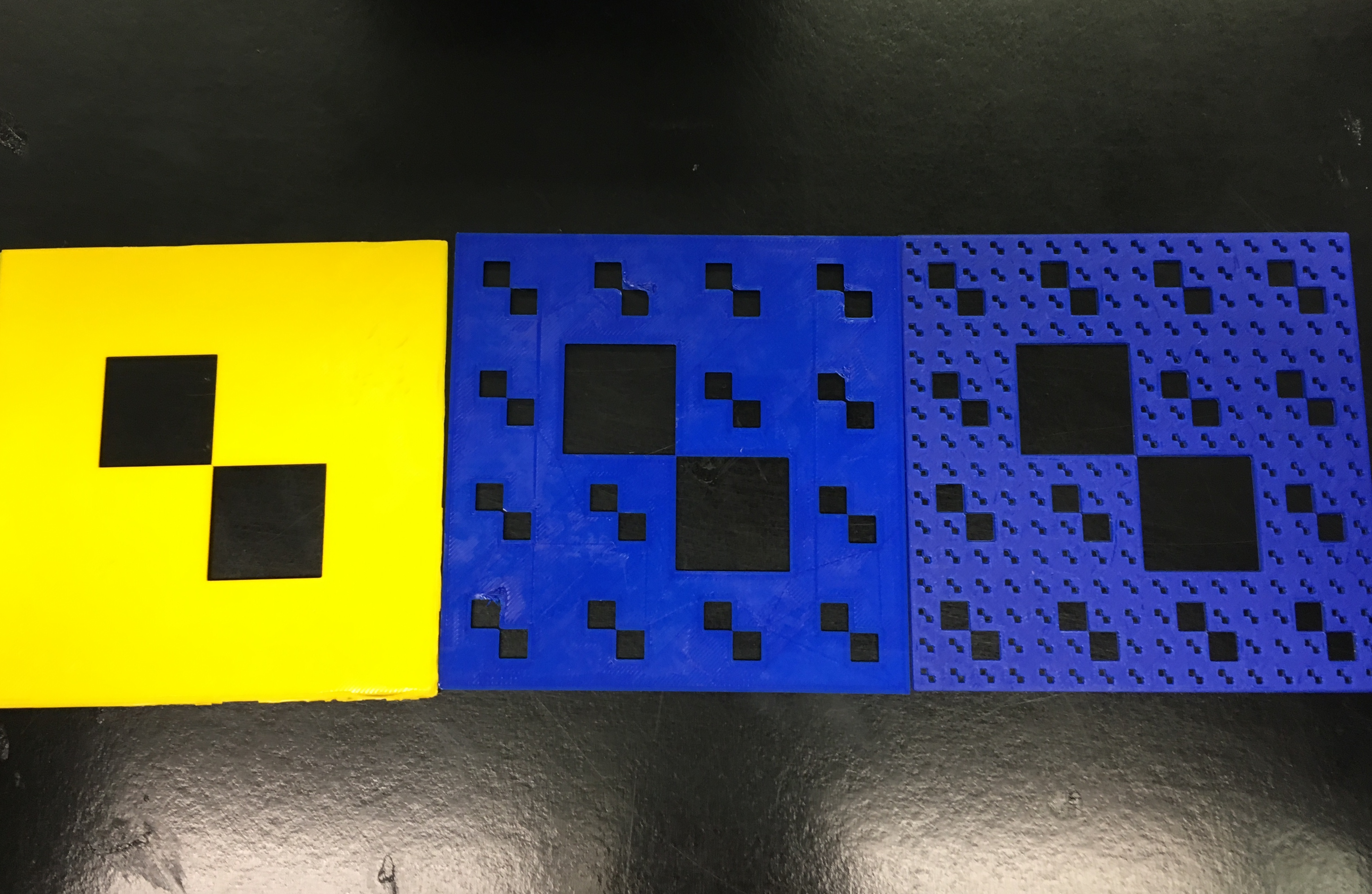# Checkerboard Carpet FractalThe fractal that I designed, aka the Checkerboard Carpet Fractal, is a simple 4×4 square with 2 pieces removed. The two coordinates that were removed were (2,1) and (1,2).

### Surface Area MATH

Length of one side is 4

Overall Shape (true for all iterations) 4^2=16

Iteration One:

2 squares with a length of 1 removed

2(1^2)=2

16-2=14

Iteration Two:

28 additional squares removed with a length of 1/4

28((1/4)^2)=1.75

16-2-1.75=12.25

Iteration Three:

392((1/16)^2)=1.53129

16-2-1.75-1.53129=10.71875

### Infinite Series MATH

Iteration One:

16-[(2*1)]=14

Solution=14

Iteration Two:

16-[(2*1)+(2*(1/16))(14)]

Solution=12.25

Iteration Three:

16-[(2*1)+(2*(1/16))(14)+(2*(1/16^2))(14)(14)]

Solution=10.71875

Formula:

a+ar^2+ar^3+ar^4+ar^5……=a(1/1-r) (if |r|<1)

Explanation:

In iteration 2, the 16 is the number of squares

The (2*1) is how many squares were removed in the first iteration (2) multiplied by the area of those boxes (1)

In the second set of parentheses, the two tells us two more boxes were removed from each of 14 more of the boxes that were not removed in iteration one. The 1/16 is the area of these smaller boxes removed.

The 14 tells us the number of times these 2 boxes were removed.

### Dimension MATH

Scaled Down by 1/4, Is a smaller copy of the whole fractal and larger one is 14 copies of this one. Iteration One Little square is 1/15th (both put together is 1/14th to total)

(1/4)^D = (1/14)

(1/(4^D)) = (1/14)

4^D = 14

log4(14) = D

D ~~ 1.90637

A^D = B

logA(B) = D